# Option Greeks Excel Formulas

This is the second part of the Black-Scholes Excel guide covering Excel calculations of option Greeks (delta, gamma, theta, vega, and rho) under the Black-Scholes model.

## Calculating Black-Scholes Greeks in Excel

I will continue in the example from the first part to demonstrate the exact Excel formulas. See the first part for details on parameters and Excel formulas for d1, d2, call price, and put price.

Here you can find detailed explanations of all the Black-Scholes formulas.

Here you can see how everything works together in Excel in the Black-Scholes Calculator.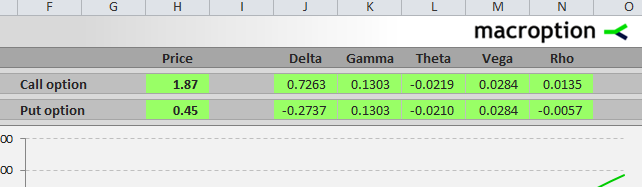## Delta in Excel

Delta is different for call and put options. The formulas for delta are relatively simple and so is the calculation in Excel.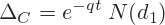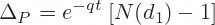I calculate call delta in cell V44, continuing in the example from the first part, where I have already calculated the two individual terms in cells M44 and S44:

``=M44*S44``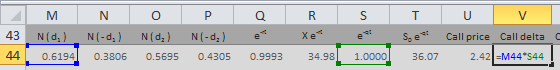The calculation of put delta is almost the same, using the same cells. Just add minus one and don't forget the brackets:

``=S44*(M44-1)``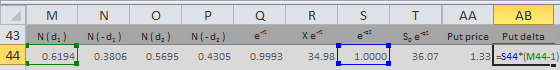## Gamma in Excel

The formula for gamma is the same for calls and puts. It is slightly more complicated than the delta formulas above: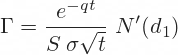Notice especially the second part of the formula: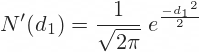You will find this term in the calculation of theta and vega too. It is the standard normal probability density function for -d1. In Excel the formula looks like this:

``=EXP(-1*POWER(K44,2)/2)/SQRT(2*PI())``

... where K44 is the cell where you have calculated d1 (see first part).

Alternatively, you can use the NORM.DIST Excel function, which I have also explained in the first part. The only difference from the first part is that the last parameter (cumulative) is now FALSE. Don't forget the minus sign before K44:

``=NORM.DIST(-K44,0,1,FALSE)``

These two formulas must return the same result.

In the example from the Black-Scholes Calculator I use the first formula. The whole formula for gamma (same for calls and puts) is:

``=EXP(-1*POWER(K44,2)/2)/SQRT(2*PI())*S44/(A44*J44)``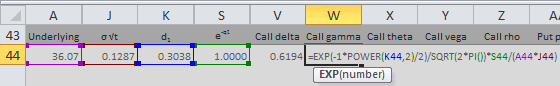## Theta in Excel

Theta has the longest formulas of all the five most common option Greeks. It is different for calls and puts, but the differences are again just a few minus signs here and there and you must be very careful. Theta is very small for many options, which makes it often hard to detect a possible error in your calculations.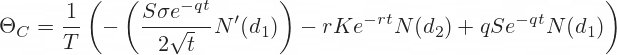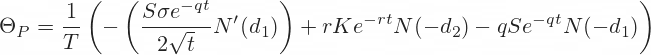Although it looks complicated, all the symbols and terms in the formulas should be already familiar from the calculations of option prices and delta and gamma above. One exception is the T at the beginning of the formulas.

T is the number of days per year. You can choose either calendar days (T=365 or 365.25) or trading days (T=252 or something similar, depending on where you trade). Based on your selection, the interpretation of theta will then be either option price change in one calendar day or option price change in one trading day.

### Call Option Theta

The whole formula for call theta in our example is in cell X44. It is long and uses several (10) other cells, but there is no high mathematics:

``=(-(A44*EXP(-1*POWER(K44,2)/2)/SQRT(2*PI())*C44*S44/(2*SQRT(G44)))-(D44*R44*O44)+(E44*A44*M44*S44))/IF(\$C\$20=2,'Time Units'!\$D\$4,'Time Units'!\$D\$3)``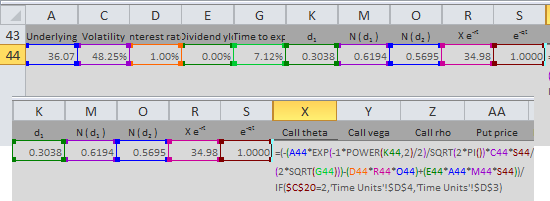The last line of the formula in the screenshot above is the T. Cell C20 in the calculator contains a combo where users select calendar days or trading days. Cells D3 and D4 in the sheet Time Units contain the number of calendar and trading days per year. If you want to keep it simple, you can replace the whole last line of the formula with a fixed number, such as 365.

You can again find the explanation of all the individual cells in the first part or see all these Excel calculations directly in the calculator.

### Put Option Theta

Analogically to call theta, the formula for put theta in cell AD44 is:

``=(-(A44*EXP(-1*POWER(K44,2)/2)/SQRT(2*PI())*C44*S44/(2*SQRT(G44)))+(D44*R44*P44)-(E44*A44*N44*S44))/IF(\$C\$20=2,'Time Units'!\$D\$4,'Time Units'!\$D\$3)``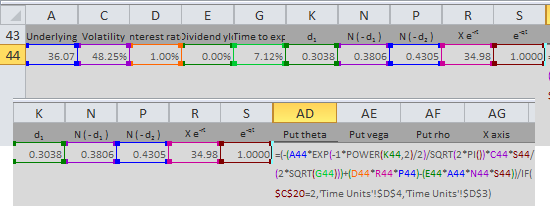## Vega in Excel

The formula for vega is the same for calls and puts: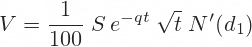There is nothing new. You can again see the familiar term at the end.

In the calculator example I calculate vega in cell Y44:

``=EXP(-1*POWER(K44,2)/2)/SQRT(2*PI())*S44*A44*SQRT(G44)/100``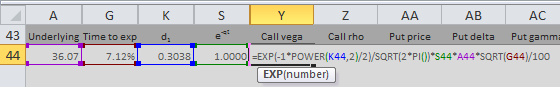## Rho in Excel

Rho is again different for calls and puts. There are two more minus signs in the put rho formula.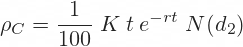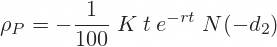In the calculator example I calculate call rho in cell Z44. It is simply a product of two parameters (strike price and time to expiration) and cells that I have already calculated in previous steps:

``=B44*G44*Q44*O44/100``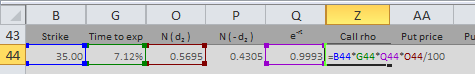I calculate put rho in cell AF44, again as product of 4 other cells, divided by 100. Make sure to put the minus sign to the beginning:

``=-B44*G44*Q44*P44/100``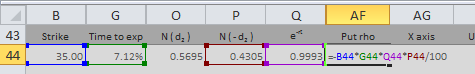## More about Option Greeks in Excel

You can also use Excel and the calculations above (with some modifications and improvements) to model behaviour of individual option Greeks and option prices in different market situations (changes in the Black-Scholes model parameters). That is beyond the scope of this guide, but you can find it in the Black-Scholes Calculator.

By remaining on this website or using its content, you confirm that you have read and agree with the Terms of Use Agreement.

We are not liable for any damages resulting from using this website. Any information may be inaccurate or incomplete. See full Limitation of Liability.

Content may include affiliate links, which means we may earn commission if you buy on the linked website. See full Affiliate and Referral Disclosure.

We use cookies and similar technology to improve user experience and analyze traffic. See full Cookie Policy.